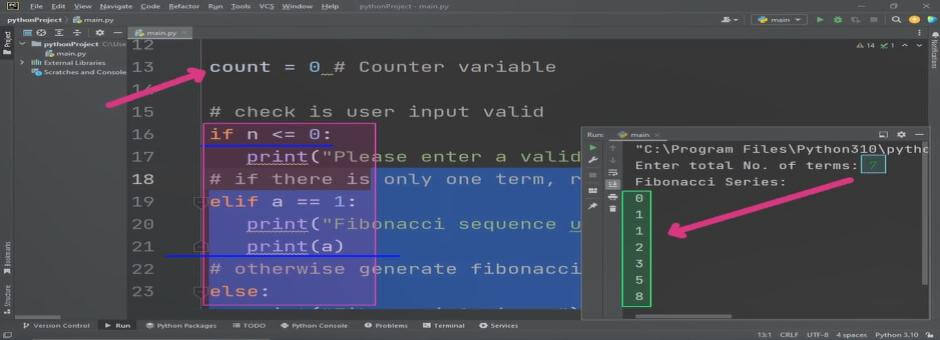# Fibonacci Series in python

## Source Code:

# Fibonacci Series Program to Print Numbers
# from series using user input

n = int(input("Enter total No. of terms: "))

# first and second term
a = 0
b = 1
# we can also write as a, b = 0, 1

count = 0 # Counter variable

# check is user input valid
if n <= 0:
# if there is only one term, return a
elif a == 1:
print("Fibonacci sequence upto",a,":")
print(a)
# otherwise generate fibonacci series
else:
print("Fibonacci Series: ")
while count < n:
print(a)
x = a + b
# Assign variables new values (Next values)
a = b
b = x
count = count + 1

## Output:

The output of above code will be as:## Working:

Fibonacci series is a mathematical sequence in which each coming number is the sum of its previous two numbers. As we say that sum of previous two numbers so that we have to specifiy first two numbers in advance so that the new number can be calculated. This is simple sequence but has its distinct importance in mathematics. This squence has also notable implementation in computer graphics.

So according to rule of sequence we declare here two variables that are called a and b. These variables store the initial two values as 0 and 1. These are also called sequence terms.

After that we declare a counter variable named as count (we can also use any other suitable name such as counter or simply c).

In this program example we are going to print specified no of terms means first we will get input from user that how many terms he want to display. Then in next step we will write a loop construct which will repeat the following algorithm until the required no of terms are not displayed.

## Fibonacci series Algorithm

1. PRINT first_term
2. new_term = first_term + second_term
3. first_term = second_term
4. second_term = new_term

When all ther terms will be displayed then loop will terminate.